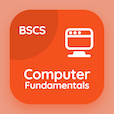Computer Science Courses

Digital Logic Design Exam Prep

Digital Logic Design Practice Test 2

# Analysis of Asynchronous Sequential Logic MCQ Questions PDF Download - 2

The e-Book Analysis of Asynchronous Sequential Logic Multiple Choice Questions and Answers (MCQs), analysis of asynchronous sequential logic quiz answers PDF chapter 2-2 to study online courses, digital logic design tests. Study Asynchronous Sequential Logic MCQ trivia questions, analysis of asynchronous sequential logic Multiple Choice Questions (MCQ Quiz) for online college degrees. The e-Book "Analysis of Asynchronous Sequential Logic MCQs" App Download: analysis of asynchronous sequential logic, circuits with latches, axiomatic definition of boolean algebra, binary code, shift registers test prep for online college classes.

The MCQ "Asynchronous circuits are useful in application where the input signals may " PDF, Analysis of Asynchronous Sequential Logic App Android & iOS (Free) with never change, change at any time, and continuously change choices for bachelor's degree in computer science. Practice asynchronous sequential logic questions and answers, Google eBook to download free sample for online computer science and engineering.

## Logic Design: Analysis of Asynchronous Sequential Logic MCQ Quiz

MCQ: Asynchronous circuits are useful in application where the input signals may

A) change at any time
B) never change
C) both a and b
D) continuously change

MCQ: The SR latch consists of

A) 1 input
B) 2 inputs
C) 3 inputs
D) 4 inputs

MCQ: Boolean algebra is also called

A) switching algebra
B) arithmetic algebra
C) linear algebra
D) algebra

MCQ: Binary coded decimal is a combination of

A) two binary digits
B) three binary digits
C) four binary digits
D) five binary digits

MCQ: Shift registers are used for

A) shifting
B) rotating
D) both a and b

### Mock Tests: Digital Logic Design Course Prep

Download Digital Logic Design Quiz App, Computer Fundamentals MCQs App and Database Management System MCQ App for Android & iOS devices. These Apps include complete analytics of real time attempts with interactive assessments. Download Play Store & App Store Apps & Enjoy 100% functionality with subscriptions!